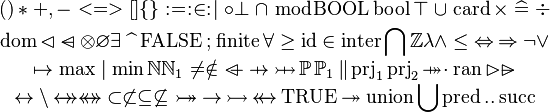# Difference between revisions of "Test area"$( ) * + , - < = > [ ] \{ \} \bcmeq \bcmin \bcmsuch \bcomp \bfalse \binter \bmod \Bool \bool \btrue \bunion \card \cprod \defi \div \atop \dom \domres \domsub \dprod \emptyset \exists \expn \False \fcomp \finite \forall \geq \id \in \inter \Inter \intg \lambda \land \leq \leqv \limp \lnot \lor \atop\mapsto \max \mid \min \nat \natn \neq \notin \ovl \pfun \pinj \pow \pown \pprod \prjone \prjtwo \psur \qdot \ran \ranres \ransub \atop\rel \setminus \srel \strel \subset \not\subset \subseteq \not\subseteq \tbij \tfun \tinj \trel \True \tsur \union \Union \upred \upto \usucc }}$ coco